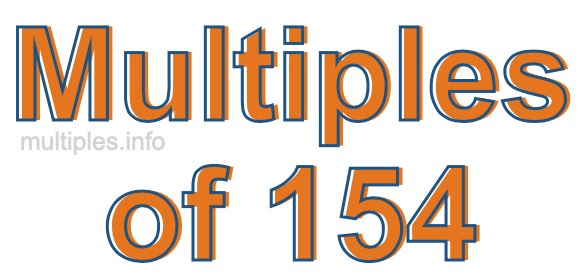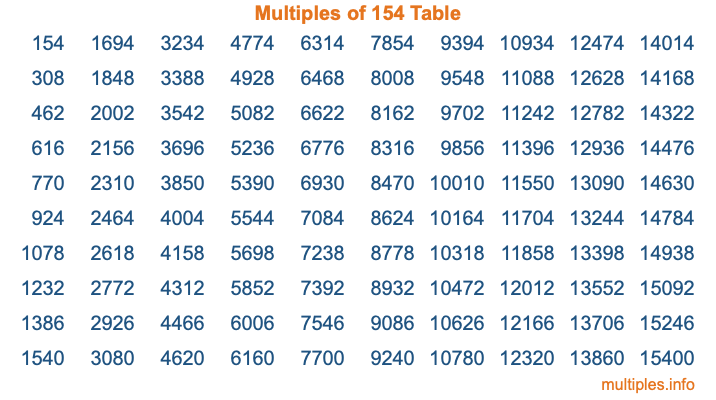Multiples of 154Welcome to the Multiples of 154 page. Here we will first teach you everything you will ever need to know about the multiples of 154, and then give you a study guide summary of everything we taught you to make sure you remember it all. Use this page to look up facts and learn information about the multiples of 154. This page will make you a multiples of one hundred fifty-four expert!

Definition of Multiples of 154
Multiples of 154 are all the numbers that when divided by 154 equal an integer. Each of the multiples of 154 are called a multiple. A multiple of 154 is created by multiplying 154 by an integer.

Therefore, to create a list of multiples of 154, you start with 1 multiplied by 154, then 2 multiplied by 154, then 3 multiplied by 154, and so on for as long as you want. Thus, the list of the first five multiples of 154 is 154, 308, 462, 616, and 770. To see a larger list of multiples of 154, see the printable image of Multiples of 154 further down on this page. We also have a category where you can choose any nth multiple of 154.

Multiples of 154 Checker
The Multiples of 154 Checker below checks to see if any number of your choice is a multiple of 154. In other words, it checks to see if there is any number (integer) that when multiplied by 154 will equal your number. To do that, we divide your number by 154. If the the quotient is an integer, then your number is a multiple of 154.

Is  a multiple of 154?

Least Common Multiple of 154 and ...
A Least Common Multiple (LCM) is the lowest multiple that two or more numbers have in common. This is also called the smallest common multiple or lowest common multiple and is useful to know when you are adding our subtracting fractions. Enter one or more numbers below (154 is already entered) to find the LCM.

Check out our LCM Calculator if you need more details about the Least Common Multiple or if you need the LCM for different numbers for adding and subtraction fractions.

nth Multiple of 154
As we stated above, 154 is the first multiple of 154, 308 is the second multiple of 154, 462 is the third multiple of 154, and so on. Enter a number below to find the nth multiple of 154.

th multiple of 154

Multiples of 154 vs Factors of 154
154 is a multiple of 154 and a factor of 154, but that is where the similarities end. All postive multiples of 154 are 154 or greater than 154. All positive factors of 154 are 154 or less than 154.

Below is the beginning list of multiples of 154 and the factors of 154 so you can compare:

Multiples of 154: 154, 308, 462, 616, 770, etc.

Factors of 154: 1, 2, 7, 11, 14, 22, 77, 154

As you can see, the multiples of 154 are all the numbers that you can divide by 154 to get a whole number. The factors of 154, on the other hand, are all the whole numbers that you can multiply by another whole number to get 154.

It's also interesting to note that if a number (x) is a factor of 154, then 154 will also be a multiple of that number (x).

Multiples of 154 vs Divisors of 154
The divisors of 154 are all the integers that 154 can be divided by evenly. Below is a list of the divisors of 154.

Divisors of 154: 1, 2, 7, 11, 14, 22, 77, 154

The interesting thing to note here is that if you take any multiple of 154 and divide it by a divisor of 154, you will see that the quotient is an integer.

Multiples of 154 Table
Below is an image of the first 100 multiples of 154 in a table. The table is in chronological order, column by column. The first column has the first ten multiples of 154, the second column has the next ten multiples of 154, and so on.The Multiples of 154 Table is also referred to as the 154 Times Table or Times Table of 154. You are welcome to print out our table for your studies.

Negative Multiples of 154
Although not often discussed or needed in math, it is worth mentioning that you can make a list of negative multiples of 154 by multiplying 154 by -1, then by -2, then by -3, and so on, to get the following list of negative multiples of 154:

-154, -308, -462, -616, -770, etc.

Multiples of 154 Summary
Below is a summary of important Multiples of 154 facts that we have discussed on this page. To retain the knowledge on this page, we recommend that you read through the summary and explain to yourself or a study partner why they hold true.

There are an infinite number of multiples of 154.

A multiple of 154 divided by 154 will equal a whole number.

154 divided by a factor of 154 equals a divisor of 154.

The nth multiple of 154 is n times 154.

The largest factor of 154 is equal to the first positive multiple of 154.

154 is a multiple of every factor of 154.

154 is a multiple of 154.

A multiple of 154 divided by a divisor of 154 equals an integer.

154 divided by a divisor of 154 equals a factor of 154.

Any integer times 154 will equal a multiple of 154.

Multiples of a Number
Here you can get the multiples of another number, all with the same attention to detail as we did for multiples of 154 on this page.

Multiples of
Multiples of 155
Did you find our page about multiples of one hundred fifty-four educational? Do you want more knowledge? Check out the multiples of the next number on our list!